# Carla

Carla is 5 years old and Jim is 13 years younger than Peter. One year ago, Peter’s age was twice the sum of Carla’s and Jim’s age. Find the present age of each one of them.

Correct result:

C =  5
J =  6
P =  19

#### Solution:

C=5
J = P-13
P-1 = 2((C-1)+(J-1))

C=5
J = P-13
P-1 = 2•((C-1)+(J-1))

C = 5
J-P = -13
2C+2J-P = 3

C = 5
J = 6
P = 19

Our linear equations calculator calculates it.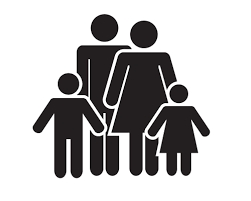We would be pleased if you find an error in the word problem, spelling mistakes, or inaccuracies and send it to us. Thank you!Tips to related online calculators
Do you have a linear equation or system of equations and looking for its solution? Or do you have quadratic equation?
Do you want to convert time units like minutes to seconds?

## Next similar math problems:

• Age problemsA) Alex is 3 times as old as he was 2 years ago. How old is he now? b) Casey was twice as old as his sister 3 years ago. Now he is 5 years older than his sister. How old is Casey? c) Jessica is 4 years younger than Jennifer now. In 10 years, Jessica will
• Lee isLee is 8 years more than twice Park's age, 4 years ago, Lee was three times as old. How old was Lee 4 years ago?
• The sum 4The sum of Robin's age is 45. Seven years ago, Robin was 16 years more than one half as old as Bruno then. How old is Bruno?
• Time passing6 years ago, Marcela's mother was two times older than her and two times younger than her father. When Marcela is 36, she will be twice as young as her father. How old are Marcela, her father, and mother now?
• Peter and PaulPeter and Paul together have 26 years. Four years ago, Paul was twice older than Peter. How much is Paul and how much Peter?
• Mother and daughter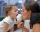Three years ago mother was three times older than daughter. After nine years she will be only twice old. How old is mother (and daughter)?
• Grandfather and grandmotherThe old mother is 5 years younger than the old father. Together they are 153 years old. How many years has each of them?
• Age questionJano is three times older than Zdeno. Zdeno is 12 years younger than Janko. How old is Jano and how old is Zdeno?
• Three siblings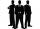Three siblings have birthday in one day-today. Together they have 35 years today. The youngest is three years younger than middle and the oldest is 5 years older than middle. How old is each?
• Mother and daughterThe mother is four times older than her daughter. Five years ago, her daughter was seven times younger than her mother. How many years do they have now?
• Three friends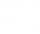Peter is eight times older than Rado, Rado is 3 times younger than Joseph. Together they have 120 years. Determine how many are each of them.
• Three brothersThe three brothers have a total of 42 years. Jan is five years younger than Peter and Peter is 2 years younger than Michael. How many years has each of them?
• Threesome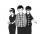Dana, Dalibor and Michael have a combined 57 years. Dana is five years older than Dalibor, but Dana is five years younger than Michael. Determine how old is Dana, Dalibor and Michael.
• Father and son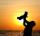Father is three times older than his son. 12 years ago father was nine times older than the son. How old are father and son?
• Triplets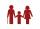Three years ago, the triplets Pavel, Petr, and Luke and their five-year-old sister Jane had together 25 years. How old is Jane today?
• Father and sonsAfter15 years will father many years as his two sons together now. There is a six-year difference between the brothers, and the older one celebrated fifty years three years ago. How old is their father now?
• AgeIn 1960 my age was equal to the digits sum of the year of my birth. What is my age now?